HOTS Questions: Polynomials

# HOTS Questions: Polynomials - Notes | Study Mathematics (Maths) Class 9 - Class 9

 1 Crore+ students have signed up on EduRev. Have you?

Q.1. If  p(x) = x2 – 2√2 x + 1, then find  p (2√2) .
Sol. Since, p(x) = x– 2 √2 x + 1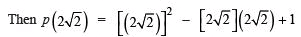= 4 (2) – 4 (2) + 1 = 8 – 8 + 1 = 1

Q.2. If  a + b + c = 9, and ab + bc + ca = 26, find a+ b2 + c2
Sol. (a + b + c)2 = (a+ b+ c2) + 2 (ab + bc + ca)
⇒ (9)= (a2 + b+ c2) + 2 (26)  = (a+ b2 + c2) + 52
⇒ a2 + b+ c2 =92– 52 = 81– 52 = 29

Q.3. Factorise :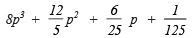Sol.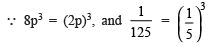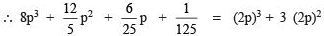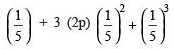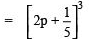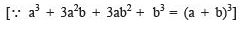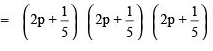Q.4. If a, b, c are all non-zero and a + b + c = 0, prove that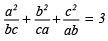Sol. ∵ a + b + c = 0  ⇒  a3 + b3 + c3 – 3abc = 0
or a+ b+ c3 = 3abc ⇒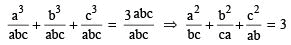Q.5. Prove that (a + b + c)3 – a3 – b3 – c3 = 3(a + b) (b + c) (c + a)
Sol.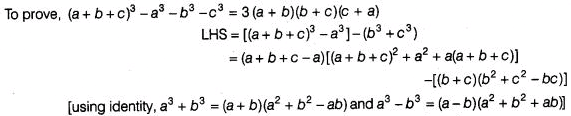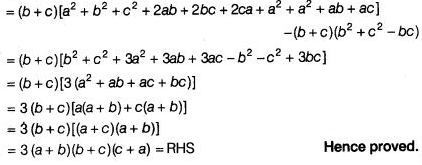The document HOTS Questions: Polynomials - Notes | Study Mathematics (Maths) Class 9 - Class 9 is a part of the Class 9 Course Mathematics (Maths) Class 9.
All you need of Class 9 at this link: Class 9

## Mathematics (Maths) Class 9

88 videos|397 docs|109 tests
 Use Code STAYHOME200 and get INR 200 additional OFF

## Mathematics (Maths) Class 9

88 videos|397 docs|109 tests

Track your progress, build streaks, highlight & save important lessons and more!

,

,

,

,

,

,

,

,

,

,

,

,

,

,

,

,

,

,

,

,

,

;